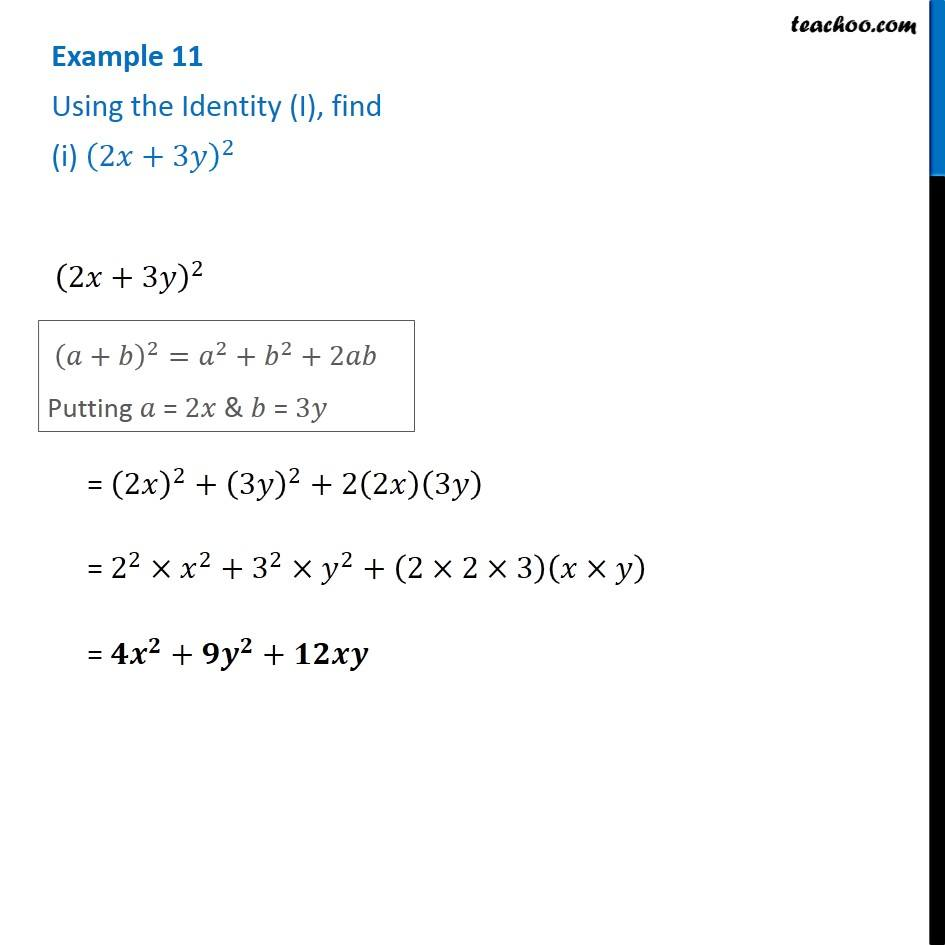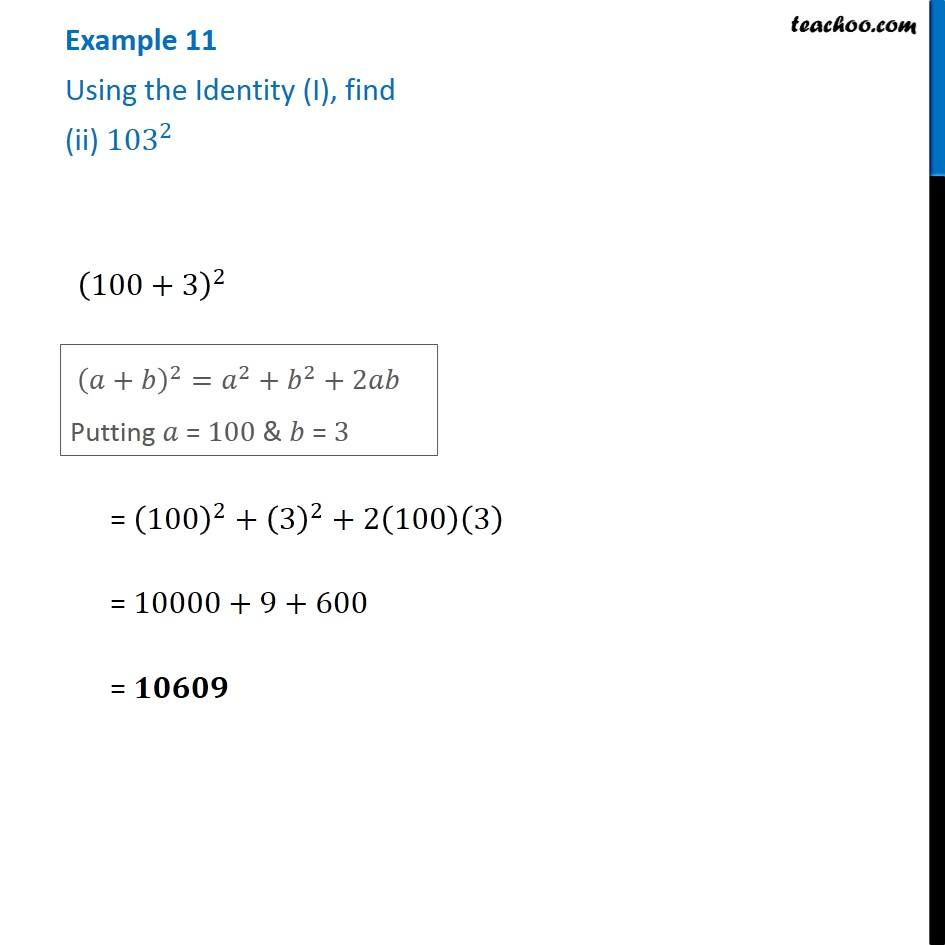1. Chapter 9 Class 8 Algebraic Expressions and Identities
2. Serial order wise
3. Examples

Transcript

Example 11 Using the Identity (I), find (i) (2𝑥+3𝑦)^2 (2𝑥+3𝑦)^2 (𝑎+𝑏)^2=𝑎^2+𝑏^2+2𝑎𝑏 Putting 𝑎 = 2𝑥 & 𝑏 = 3𝑦 (𝑎+𝑏)^2=𝑎^2+𝑏^2+2𝑎𝑏 Putting 𝑎 = 2𝑥 & 𝑏 = 3𝑦 Example 11 Using the Identity (I), find (ii) 〖103〗^2 (100+3)^2 (𝑎+𝑏)^2=𝑎^2+𝑏^2+2𝑎𝑏 Putting 𝑎 = 100 & 𝑏 = 3 = (100)^2+(3)^2+2(100)(3) = 10000+9+600 = 𝟏𝟎𝟔𝟎𝟗

Examples